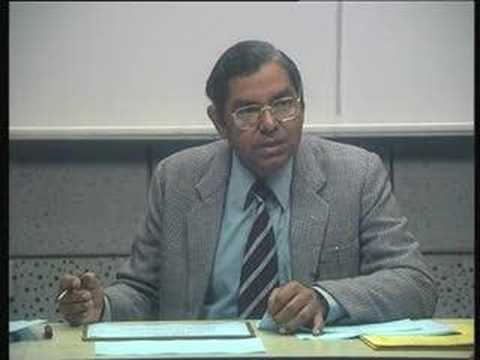Prof. Arun Kanda
Prof. Arun Kanda is very intelligent professor of Department of Mechanical Engineering, IIT Delhi. Not only on expert in mechanical engineering, he has also deep knowledge of financial and project management. Today, I saw his lecture ( 54 minutes and 40 second)  on  topic "Financial Evaluation of capital Decisions." In this lecture he explains key factors which affect the decision of investment in any capital project.

Here, we are discussing , what key factors, he discussed in his factor. Prof. Arun Kanda told that for analysis of any project financially, we have to calculate NPV, IRR and also payback period. All these are the best tool for comparing different project. If NPV is higher, it means this project is more valuable for us. If  IRR is higher, that project is best for us. We also analyze the payback period. Short payback period project is better than long payback period project.

Prof. Arun Kanda also explains that cash inflow is just like revenue of each year. If we have to calculate NPV, we should know revenue after tax. For calculating revenue after tax, we have to calculate depreciation. Profit after deducting depreciation can be used for calculating tax. Then after calculating tax, we calculate profit after tax but before depreciation. Suppose, our profit is 10,000 and depreciation is Rs. 100. Balance of profit will be 9900. Now, tax is calculated on this 9900. Suppose tax rate is 10% and then tax is Rs. 990. Now we will deduct tax from the profit before depreciation.

* {Note : Depreciation is not cash outflow. So, it will not decrease cash profit amount. }

Profit after tax but before profit will be 10000 - 990 = 9010. This will be used for calculating of NPV.

Prof. Arun Kanda explains each tool of evaluating financial project in presentation form. Way of providing lecture is very nice..

## \$type=three\$a=hide\$cm=hide\$s=hide\$show=/2019/06/10-steps-to-become-better-investor.html\$l=hide

Name

false
ltr
item
Accounting Education: Lecture of Prof. Arun Kanda on Financial Evaluation of a Project
Lecture of Prof. Arun Kanda on Financial Evaluation of a Project
http://i2.ytimg.com/vi/qJCni5HOQx4/hqdefault.jpg
Accounting Education
http://www.svtuition.org/2010/11/lecture-of-prof-arun-kanda-on-financial.html
http://www.svtuition.org/
http://www.svtuition.org/
http://www.svtuition.org/2010/11/lecture-of-prof-arun-kanda-on-financial.html
true
2410664366776677676
UTF-8